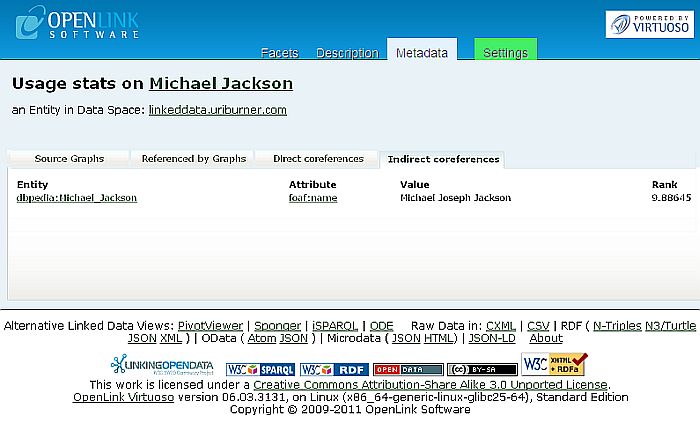### 16.11.6.Usage Statistics

1. Use the Faceted Browser Search and Find User Interface to search for information on "Michael Jackson":

Figure16.138.Usage Statistics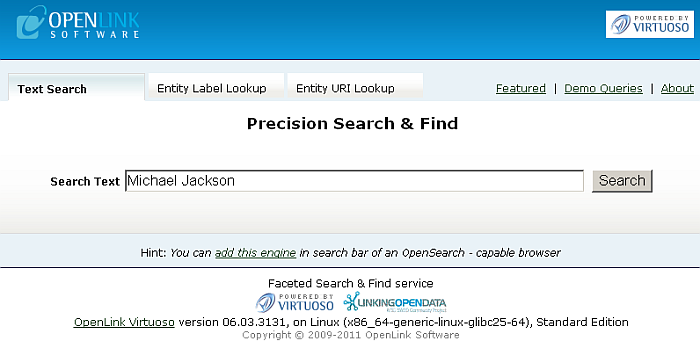2. Results of the following form should be returned for the network resource data being fetched.

Figure16.139.Usage Statistics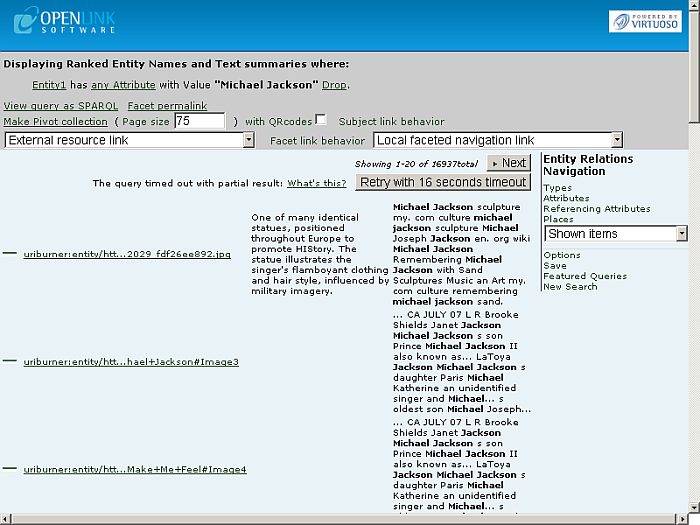4. Results about "Michael Jackson" as Type/Label/Count list should be displayed:

Figure16.140.Usage Statistics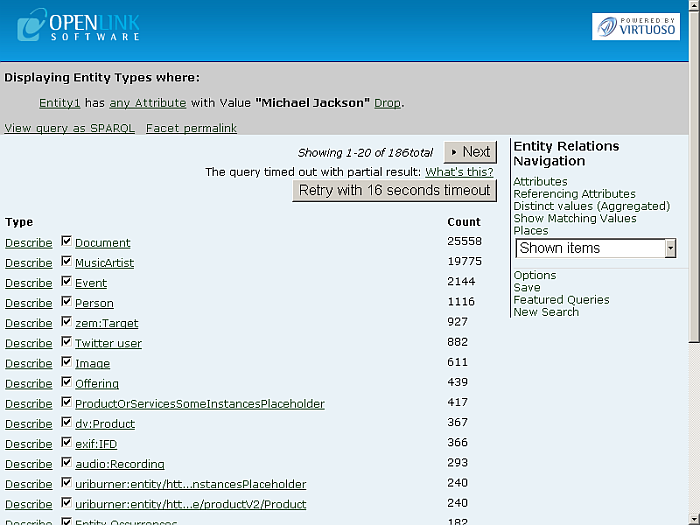5. You can navigate amongst the search results pages by using the "Prev" and "Next" buttons. Click for ex. "Next":

Figure16.141.Usage Statistics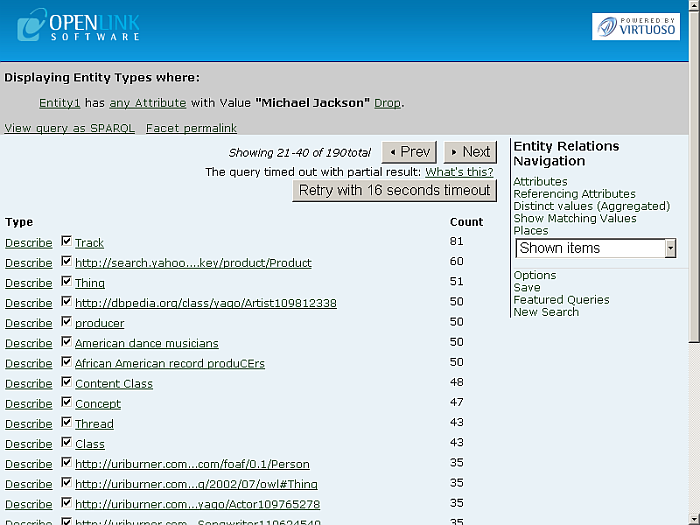6. Click a type link, for ex.:

```http://dbpedia.org/class/yago/Artist109812338
```
7. Should be shown type results and:

```Displaying Ranked Entity Names and Text summaries where:

Entity1 has any Attribute with Value "Michael Jackson" Drop.
Entity1 is a yago:Artist109812338 . Drop
```

Figure16.142.Usage Statistics```dbpedia:Michael_Jackson
```
9. Results about "Michael Jackson" as Attribute/Value list should be presented:

Figure16.143.Usage Statistics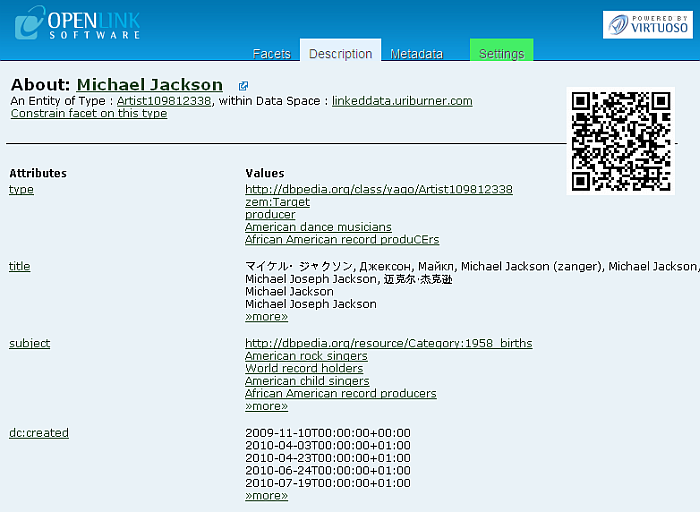Figure16.144.Usage StatisticsFigure16.145.Usage Statistics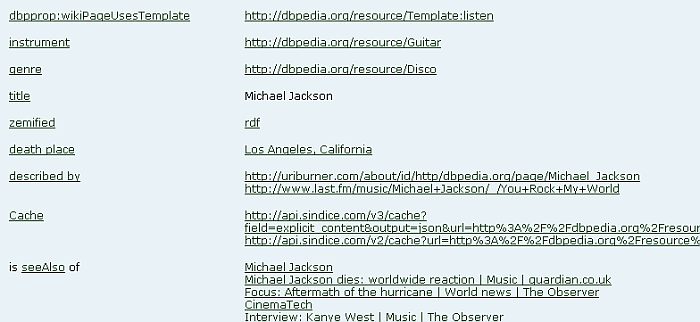Figure16.146.Usage Statistics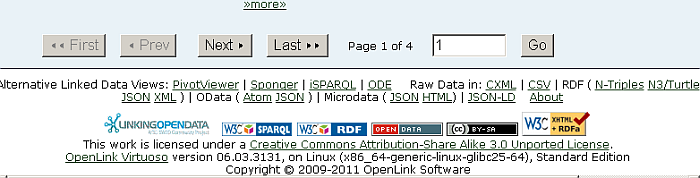10. You can navigate amongst the search results pages by using the "First", "Prev", "Next" and "Last" buttons. Click for ex. "Last":

Figure16.147.Usage Statistics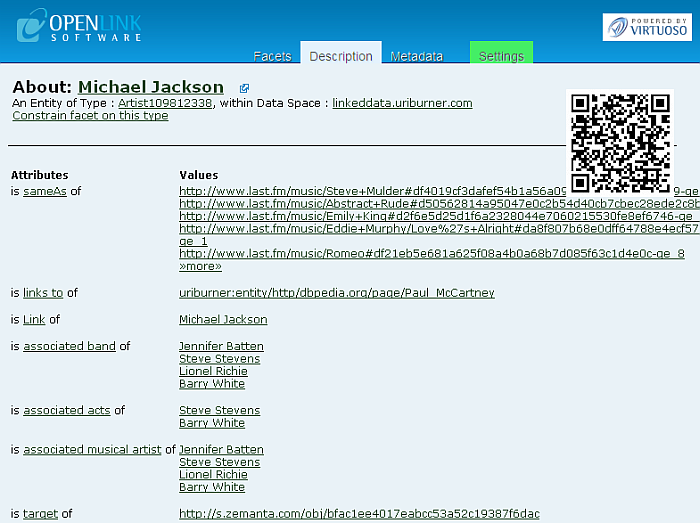Figure16.148.Usage Statistics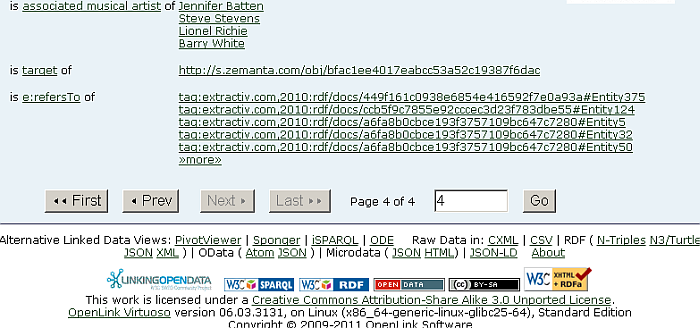12. Results of usage statistics for "Michael Jackson" grouped in 4 tabs should be shown:

1. Referenced by Graphs: shows how many times the URI is found as subject in the relevant graph(s):

```SPARQL
SELECT ?g count (*)
where
{
graph ?g { <URI> ?p ?o }
}
group by ?g
order by desc 2
limit 20
```

Figure16.149.Usage Statistics2. Source Graphs: shows how many times the URI is found as object in the relevant graph(s):

```SPARQL
SELECT ?g count (*)
where
{
graph ?g { ?s ?p <URI>  }
}
group by ?g
order by desc 2
limit 20
```

Figure16.150.Usage Statistics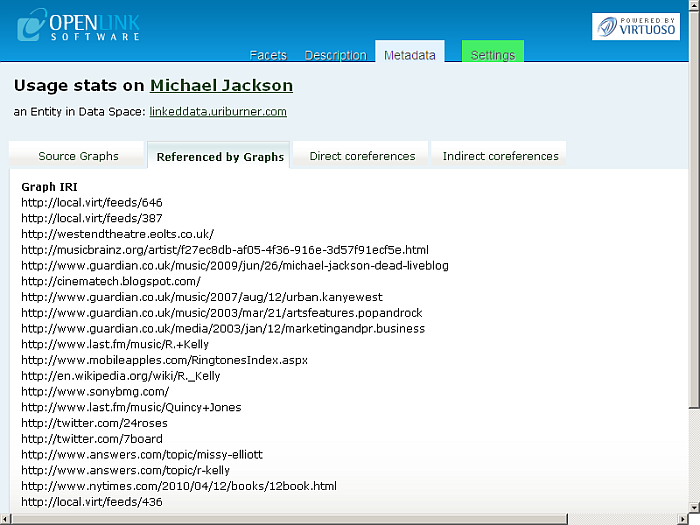3. Direct co-references: shows results as subject and calculated rank, based on running transitive closure over owl:sameAs of the URI in subject or object:

```SPARQL
SELECT ?syn ( sql:rnk_scale (<LONG::IRI_RANK> (?syn)))
where
{
{ SELECT ?s ?syn
where
{
{?syn owl:sameAs ?s } union {?s owl:sameAs ?syn}
}
}
option (transitive, t_distinct, t_min (0), T_in (?s), t_out (?syn)) . filter (!isliteral (?syn) &amp;&amp; ?s = <URI> )
}
order by desc 2
limit 20
```

Figure16.151.Usage Statistics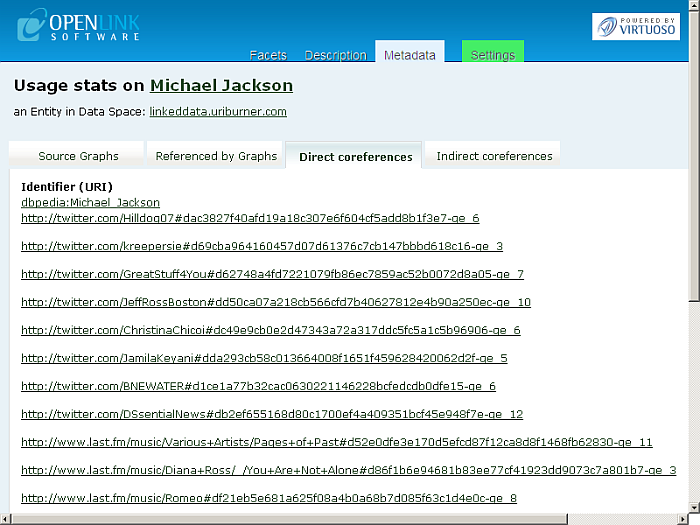4. Indirect co-references: shows expanded results for objects concur with the URI by IFP:

```SPARQL
SELECT distinct ?syn ?p ?o (sql:rnk_scale (<LONG::IRI_RANK> (?syn)))
where
{ <URI> ?p ?o .  filter (0 != (<LONG::bif:rdf_is_sub> ("b3sifp", ?p, lod:ifp_like, 3))) .
?syn ?p ?o .
}
order by desc 4
limit 20
```

Figure16.152.Usage Statistics